# How To Calculate Time in Google Sheets

Whether you’re looking to throw together a quick financial spreadsheet or you want to work together with a co-worker on an Excel-like document, Google Sheets is a great, web-based, and free alternative to Excel, an app used by millions around the country to load data into a document, view and share it, and use math to track specific content with a spreadsheet.

One of the most useful aspects of spreadsheet programs is how flexible they are. A spreadsheet can serve as a database, as a calculation engine, as a platform on which to do statistical modeling, as a text editor, as a media library, as a to-do list, and on and on. The possibilities are nearly endless. One particularly common use for spreadsheets, including Google Sheets, is for tracking time such as hourly employee time schedules or billable hours.

If you are using Google Sheets to track time in this way, then you will frequently find yourself needing to calculate the difference between two timestamps, that is, the amount of time that passed between two time events. For example, if someone clocked in at 9:15 AM and then clocked out at 4:30 PM, they were on the clock for 7 hours, 15 minutes. If you need to use Sheets for something like this, you’ll quickly notice that it is not built to handle these kinds of tasks.

Still, while Google Sheets is not designed specifically to handle functions like this, it is easy to persuade it to do so with a little preparation. In this article, I will show you how to automatically calculate the difference between two timestamps in Google Sheets.

For this article I will use a timesheet format, showing the time the person started work, the time they left, and a (calculated) duration. You can see the spreadsheet I used for this example below:

## Calculating Time in Google Sheets

Measuring the difference between two cells containing time data requires that Sheets understands that the data in the cells is time data. Otherwise, it will calculate the difference between 9:00 AM and 10:00 AM as 100 rather than 60 minutes or one hour.

To do this, the time columns need to be formatted as Time and the duration column needs to be formatted as Duration. Follow the steps below to set up your spreadsheet:2. Select the first (Time In) time column and click the ‘123’ format drop-down in the menu, then select Time as the format.3. Repeat for the second (Time Out) time column.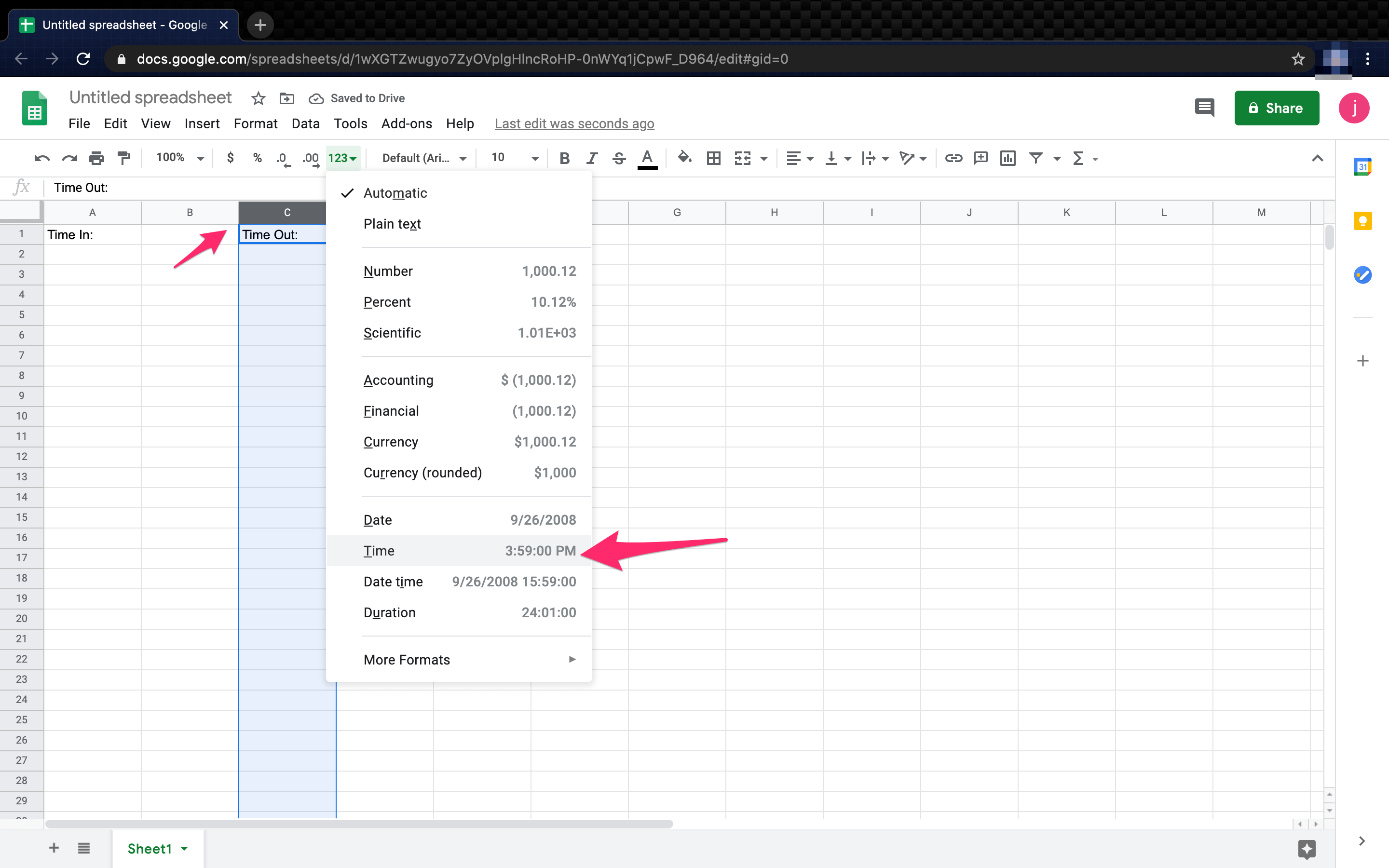4. Format the Hours Worked column as Duration in the same way.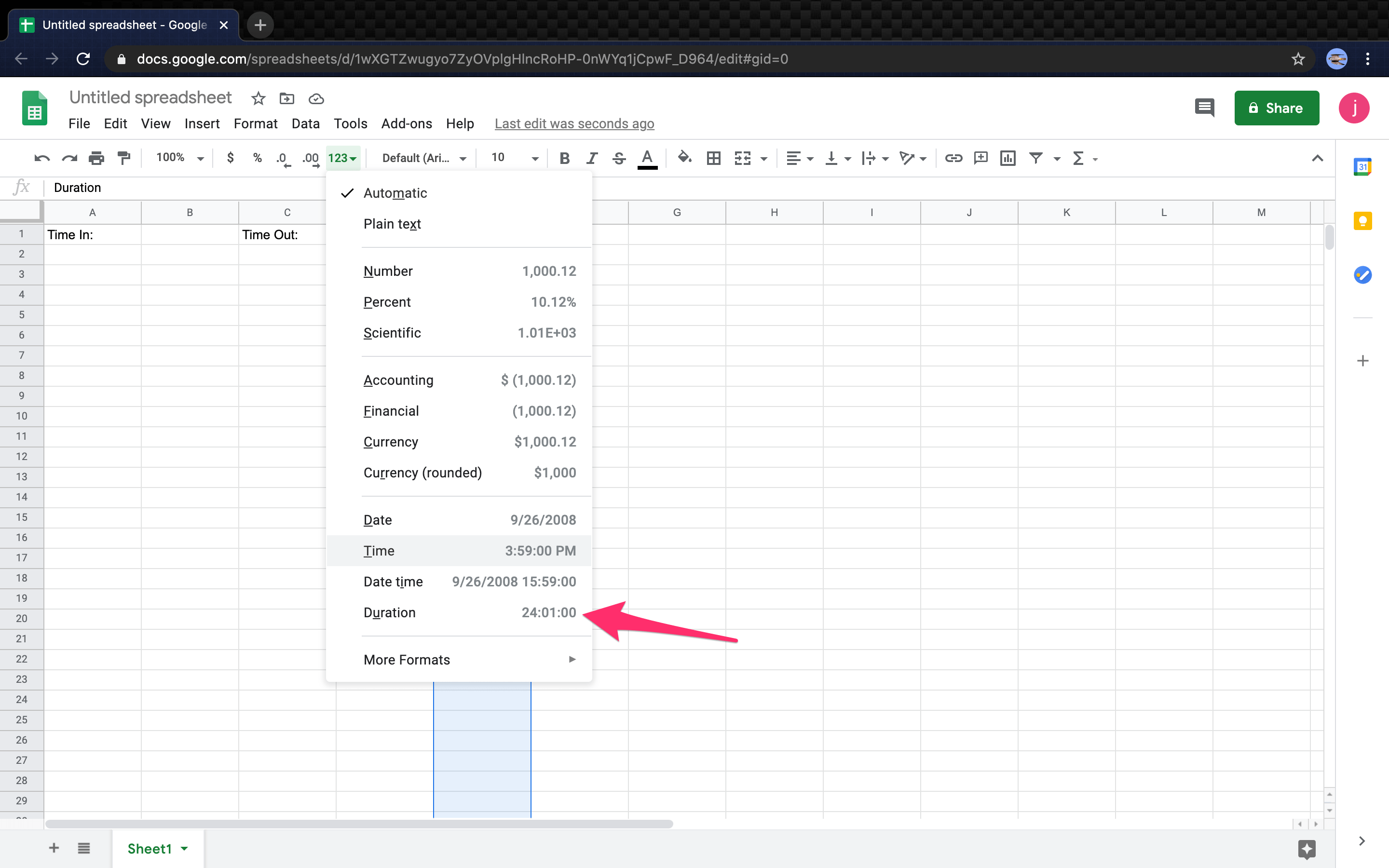5. Now the columns are formatted correctly to calculate elapsed time between two recorded timestamps.

In our example, Time In is in column A, beginning at A2, and Time Out is in column C, beginning at C2. The hours worked time is in column E. With the formats set correctly, doing the calculation couldn’t be easier. All you need to do is use the following formula: ‘=(C2-A2)’. This will give you the elapsed time between the two cells and display it as hours.

You can take this calculation further by adding dates too. This is useful if you have work shifts that go more than 24 hours or that include two days within a single shift. To do this, set the time in and time out columns to be Date Time format.

That’s all there is to it. By following the steps listed above and using the provided formula, it is incredibly easy to calculate time in Google Sheets.

There’s much you can do with Google Sheets, here are some of the most asked questions.

### How Do You Add Breaks When Calculating Time?

When calculating the hours worked by employees, it’s helpful to know how to add breaks to your time sheet. There are several ways to add a lunch break into the hours worked by employees, we’ll cover the break start and end method.

1. Create the Break Start Column and add all of the breaks into the cells.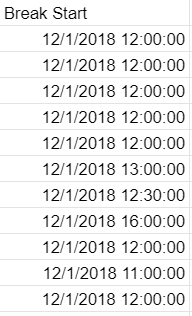You can just leave the column format on automatic, Google Sheets will do the rest.

2. Next, create the Break End Column and leave the format on automatic.3. Calculate the hours for the Hours Worked Column. So, E2 = (B2-A2) + (D2-C2). Which is, (Break Start – Time Start) + (Time Out – Break End) = Hours Worked for the day.Calculate that for every row, so that your Hours Worked Column looks like this.So, E3 = (B3-A3) + (D3-C3), etc.

### How Do You Convert Minutes to Fractions?

When dealing with increments of time, it might be useful to be able to convert them into fractions instead of minutes, i.e. 30 minutes = 1/2. Converting minutes to fractions is easy, there are several ways to accomplish this.

1. Create a new cell, K2 in this example, and format it to Number.2. Set the formula to ‘= (E2) * 24‘.If you followed along, the total should be 5.50 and will look something like this.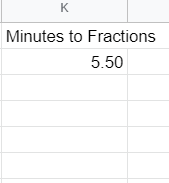You can easily apply this to a group or column of cells and convert them in a matter of seconds.

### How Do You Find the Shortest Amount of Time Worked?

If you’re in need of quickly locating the least amount of time worked, then this should help. The MIN() function is a built-in function that allows you to quickly locate the minimum value in a list of numbers.

1. Create a new cell and set it to Duration, I2 in this example, and assign it the function ‘=MIN(E2:E12)‘.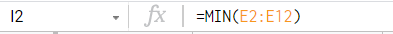Assuming you followed the example, the Min Hours Worked Column should be 5:15:00.You can easily apply the MIN() or MAX() function to a column or group of cells, give it a try for yourself.

### How Do You Calculate the Total Hours Worked?

If you’re not familiar with programming or Excel, then some of the built-in functions for Google Sheets may seem strange. Luckily, it doesn’t take much calculate the total hours worked. In this example, we’ll calculate the total hours worked by all the employees in a day.

1. Create a new cell and assign it as Duration, in this example cell G13.2. In the Formula (fx) Bar: enter ‘=SUM(E2:E12)‘. This will give you the total hours worked from cells E2 through E12. This is standard syntax for Excel and various programming languages.The total should be 67:20:00 and look like this:## Final Thoughts

Google Sheets wasn’t specifically designed to be used as a timesheet but can be easily configured to do just that. This simple setup means you can track hours worked quickly and easily. When time-spans cross over the 24-hour mark, things become a little more complicated, but Sheets can still pull it off by changing from Time to Date format.

Hopefully, you found this tutorial helpful. If you did, make sure to check out more TechJunkie articles to find the tips and tricks you need to make the most out of your favorite technological tools and applications.

(Want to figure out a person’s age? Check our tutorial on how to calculate age from a birthdate in Sheets. You can also read our article on figuring out how many days have passed between two dates in Sheets, or maybe you want to learn how to display today’s date in Sheets.)

Got any other time tracking tips for Google Sheets? Tell us about them below!

## 20 thoughts on “How To Calculate Time in Google Sheets”ab says:
Cannot make this work. Column C (minus) says “waiting for number, but is text” whatever I choose for formats. Could you share your sheet?emma says:
How do i take away breaks – ie time started, break, time finished on a google sheet?Anna says:
When I format the column as Time it changes all the numbers to something weird and unhelpful. What am I doing wrong?Christopher Meeker says:
I have an issue similar to this but not exactly. I want to track swim times inside Google Sheets. Specifically, I would like to have an array of past swim times for each event with format (mm:ss.ms) and use the ‘MIN’ function to pull out the minimum value. I have tried to type the values and create a custom ‘duration’ number for the fields to no avail. I can’t get Google Sheets to see the typed in values as numbers for the MIN function to work properly.
Example (mm:ss.ms) format:
B2 = 0:32.06, C2 = 0:31.60, D2 = 0:31.78
=MIN(B2:D2) >> Returns 0
Am unable to get Google Sheets to parse the “duration” cells properly or I do not understand how it does parse them more likely.Morgan says:
is there a way to do this the other way around? So say something starts at 6 and lasts 30 mins, is there a way to have the next time say 6:30 because of that?Jennifer says:
Is there a way to change the totals to fractions? Instead of 15 minutes to give you .25?Kevin James Bowman says:
I know you left this over a month ago, so I am leaving it for the next person. This will give you the

=(HOUR(C2)+(Minute(C2)/60)-(HOUR(A2)+((Minute(A2)/60))))Jennifer Duff says:
It worked! Thanks so much. I had just been writing them into a column all month.Eileen says:
I have an issue with adding up a months total hours, I have for instance 4 weeks where some people have worked 9 hours per day, that adds up correctly. But then i have someone else for the 4 weeks that has worked 9 hours a day, but on 4/5 days he worked 14 hours, but his hours add up to less than the guy that worked 9 hours straight????? the total hours is setup as durationHarry Wilcock says:
Hi there Sheets Genius’s, so ive managed to get the formula to work but how do I say multiply my hours worked figure by an hourly rate, because ie: 0.45.00 minutes multiplied by say \$30.00/h just gives me \$0.94, kinda stuck on this not sure how to get it to work so I get 0.75 of an hour x by hourly rate, I need the hours worked to be in a percentage in the formula argh spreedsheets! Any help would be great thanksLacey says:
Hi Harry,

I had the same problem as you and I think I figured it out. If you change your total hours worked cell to a number format and then multiply the number in that cell by 24, it should give you the correct hours in a plain number format that you can then multiply by the hourly rate.

Hopefully this works for you!

LaceyTerence says:
Hi, How can I find the shortest time in a google sheets time table ?Steve says:
After setting duration of time worked between Start and End times, is there a way to Subtract 30 minutes (for lunch) in the code? or will that need to be done seperately?C says:
I’m trying to do the same…Eileen says:
I add a lunch colomn and put the lunch hour in as 01:00 and it deducts the 1 hour.Randy says:
Is there a way to have timestamps from a google form automatically populate the spreadsheet side by side much like the example above:
Time In Time out Hours worked
1/12/2019 16:00:42 1/12/2019 17:00:42 1:00subhradip sinha says:
HOW to calculation total of working hours in Docs sheetAlyssa says:
How can I add another column to calculate a lunch break?Vanessa Plett says:
I put in 3:15 pm until 4:10 pm and it always clocks it as 12:55 min If I go 3:15-4:15 it is 1 hour Why is that?Vanessa Plett says:
I figured it out. I have to change the formula to duration format.Dillon says:
I want it to display the percentage of the hour, for instance :15 = .25 hours. so instead of having the “duration” display “8:15:00” I want it to display “8.25”. Anyone figure out how to do this?David says:
The delta value is in units of days: 24 hours = 1.0. So format the delta cells not as time but as numbers with 2 digits past the decimal, and change the formula to divide by 24, e.g., (B1-A1)/24.David says:
Oops, that should be *24. not /24!L says:
I have a similar setup but instead of calculating duration, I have a known start time and want to add an expected (given) duration to that to come up with an expected end time. I can’t seem to get Sheets to add a given duration to a time. Anyone know how?David Olsson says:
If you have the duration in a cell, format that cell as Duration (>Format>Number>Duration) and enter the duration as h:m:s. You can then use that cell in arithmetic formulas with date/time values. Alternatively you could I think you could use the TIMEVALUE function to get the fraction of a day equivalent to a give time string and use that value in your formula. I didn’t do it that way.

Disclaimer: Some pages on this site may include an affiliate link. This does not effect our editorial in any way.

Todays Highlights